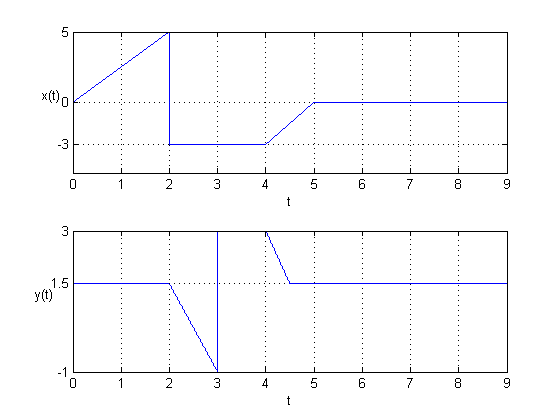# Express y(t) as a function of x(t)

• hogrampage

## Homework Statement

Express y(t) as a function of x(t).

https://www.physicsforums.com/attachment.php?attachmentid=61309&d=1378005939

## The Attempt at a Solution

Transformations:
-x(t)
0.5x(t)
x(2t)
x(t-2)
x(t)+1.5

∴y(t) = -0.5x(2t-4)+1.5

Here is the plot:As seen in the plot above, it is different from the figure in the book (P2.4(b)) on the time interval 4 < t < 5. Where am I going wrong?

Thanks!

Last edited: